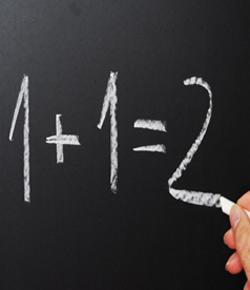# Ks2 Maths Diagnostic Primary

20 Questions | Attempts: 852SettingsDesigned to assess levels of pupils using app primary objectives from levels 2c to 3a

• 1.
• A.

A

• B.

B

• C.

C

• D.

D

• 2.
• A.

a = triangle b= cylinder c = hexagon d = rectangle

• B.

a = triangle b = hexagon c= rectangle d= cylinder

• C.

a = triangle b = pentagon c= square d = cylinder

• D.

a = triangle b = hexagon c= square d= cylinder

• E.

a = triangle b = pentagon c= square d = cuboid

• 3.
• A.

A

• B.

B

• C.

C

• D.

D

• 4.
• A.

1

• B.

2

• C.

3

• D.

4

• E.

5

• 5.
Put these numbers in order, from the lowest to the highest81 ,              24,               72,               14,                54
• A.

14, 24, 72, 54, 81

• B.

14, 54, 24, 72, 81

• C.

14, 24, 54, 72, 81

• D.

14, 54, 24, 81, 72

• E.

72, 54, 24, 81, 14

• 6.
Whats half of      18?
• A.

6

• B.

5

• C.

9

• D.

10

• E.

8

• 7.
If     3  +   7  =   10 Whats     30   +    70  =
• A.

100 because you just put extra zeros after the numbers

• B.

10 looks the same

• C.

• D.

90

• E.

120

• 8.
• A.

£0.805

• B.

£5.80

• C.

£5.85

• D.

£0.85

• E.

£5.805

• 9.
350   divided by    10   =
• A.

3500

• B.

3.5

• C.

0.35

• D.

3500

• E.

35

• 10.
1/2    =   same as
• A.

3/4

• B.

2/4

• C.

3/5

• D.

4/6

• E.

5/7

• 11.
7  x   10   =   82   -   [   ]
• A.

20

• B.

32

• C.

15

• D.

12

• E.

18

• 12.
49 divided by  3 ?
• A.

19 remainder 1

• B.

12 remainder 2

• C.

17

• D.

21

• E.

16 remainder 1

• 13.
A sweet costs 10 p.  A pencil costs 20p.  How much does a pencil and a sweet cost?
• A.

25 pence

• B.

30 pence

• C.

18 pence

• D.

21 pence

• E.

25 pence

• 14.
Compare the numbers 14 and 7 ?  How much larger is 14 than 7?
• A.

7 times larger

• B.

Twice as large

• C.

Half as large

• D.

• E.

Difficult to say

• 15.
Find the next number in the pattern   5,       8,       11,       14, ....
• A.

15

• B.

21

• C.

18

• D.

17

• E.

19

• 16.
The following addition was shown in a text book.27 +    45  =    62Whats wrong with this answer?
• A.

Nothing

• B.

Forgotten to carry the one over from adding 7 and 5

• C.

Thats not how you add you have to take 5 away from 7 first

• D.

Should be 162

• E.

You must always add in columns

• 17.
The     sum    of    32   and  25   is
• A.

57

• B.

47

• C.

36

• D.

55

• E.

59

• 18.
56 -  10 = 46If 10 is increased to 12, how would this change the answer?
• A.

The answer would be the same

• B.

• C.

• D.

14

• E.

8

• 19.
Five friends have names,    Andy, Fred, Rebecca, Aisha and TomHow many people have names less than 5 letters long
• A.

4

• B.

0

• C.

1

• D.

2

• E.

3

• 20.
What is the probability of  seeing two suns shine tommorrow?
• A.

Uncertain

• B.

Certain

• C.

Impossible

• D.

Unlikely

• E.

Very likely

## Related TopicsBack to top
×

Wait!
Here's an interesting quiz for you.